# Turtle Program Images

As a regular viewer of Numberphile videos, the last one included a subtle note which caught my attention. At the end of the video about Plotting Pi and Searching for Mona Lisa, Matt asks if somebody could send him a number that draws his face.

That sounds like a perfect fun project to learn something new. I wanted to have a timely solution that doesn't require sophisticated optimizations. Turtle programming describes the setting, where a picture is described by an alternating sequence of distances and turning angles.

Info

For a better explanation, I recommend watching both videos by Matt on Numberphile:

## Overview

As an overview, I planned the following steps

• acquire a picture of Matt
• convert it to black and white
• find a path that covers all the dark areas
• represent the path as a turtle program
• deliver

## Picture

Fortunately, Matt has a good (smallish resolution) picture on his website.I used gimp to apply a gaussian blur and then reduce the picture to a two-level black and white image.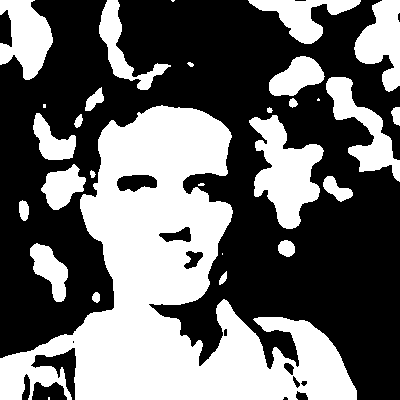Knowing the original image, the similarity is clearly visible.

There is no programmatic way for this step. It has to look nice.

## Finding a Path

Given a 2d array of bits (black or white), we need the turtle to move wherever the image is black and avoid white areas. The constraint which makes the problem interesting is, that the pen cannot be lifted to travel somewhere else.

A perfect result is impossible if the image contains more than one contiguous region, as the pen has to cross somewhere.

The following steps are all too tedious to perform by hand, so python it is.

First, we load the picture and extract the first channel (red) as it is still stored in RGB.

from PIL import Image
from numpy import asarray
import numpy as np

matt = Image.open("matthew_henderson.png");
a = asarray(myImage)
bw=a[:,:,1]


Filling an arbitrary continuous shape with a single line is quite difficult.

Note

If you are interested in perfectly filling a shape with a line, maybe this pointer helps: https://stackoverflow.com/questions/15668149/polygon-infill-algorithm

A first relaxation of the problem is to allow the line to cross an already drawn area. A crude strategy to cover all black pixels is then to use the fact that the image is a raster of pixels. We limit the turtle to always move exactly one pixel forward and then turn in 90° turns to only move on the pixel grid.

An arbitrary starting point is the lower right corner which belongs to a black region. From there the turtle searches in a diamond shape for the nearest black pixel of the picture which has not yet been colored. The find_nearest function returns the coordinates of the nearest pixel to color:

def clamp(n, minn, maxn):
return max(min(maxn, n), minn)

def find_nearest(ox,oy):
ax = ox
ay = oy
out = False
while ay > -400:
if ax <= ox and ay == oy and not out:
ax -= 1
out = True
elif ax < ox and ay >= oy:
ax += 1
ay += 1
elif ax >= ox and ay > oy:
ax += 1
ay -= 1
elif ax > ox and ay <= oy:
ax -= 1
ay -= 1
elif ax <= ox and ay < oy:
ax -= 1
ay += 1
out = False

cx = clamp(ax,0,399)
cy = clamp(ay,0,399)

if (bw - filled)[cy][cx] == 0:
return (cx,cy)


It remains to always move the turtle to the nearest uncolored pixel. The proper way would be to find a path that does not cross a white area, but that becomes surprisingly difficult. Instead, this simple version first aligns the x position and then the x generating some horizontal and vertical lines as artifacts.

With each move of the turtle, a new direction is stored as a number from 0 to 3 corresponding to right, up, left, down.

x=y=399
filled=np.zeros((400,400))
filled[x][y] = 1
with open("face.number","w") as fd:
direct = 0 # east
dc = 0
for i in range(90001):
r = find_nearest(x,y)
if r is None:
break
nx,ny = r
while x != nx:
if x > nx:
x-=1
dc = (2-direct)%4
direct = 2
#print("move left")
else:
x += 1
dc = (0-direct)%4
direct = 0
#print("move right")
filled[y][x] = 1
fd.write("%d"%dc)
while y != ny:
if y > ny:
y-=1
dc = (1-direct)%4
direct = 1
#print("move up")
else:
y += 1
dc = (3-direct)%4
direct = 3
#print("move down")
filled[y][x] = 1
fd.write("%d"%dc)

if i%10000 == 0:
print("step ",i)
p = Image.fromarray(((np.ones((400,400))-filled)*250).astype('uint8'))
display(p)


With a debug picture output every 10000 steps, we get the series of pictures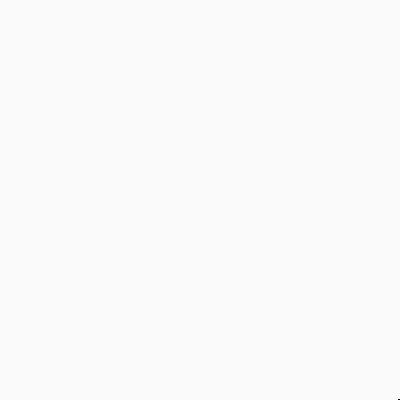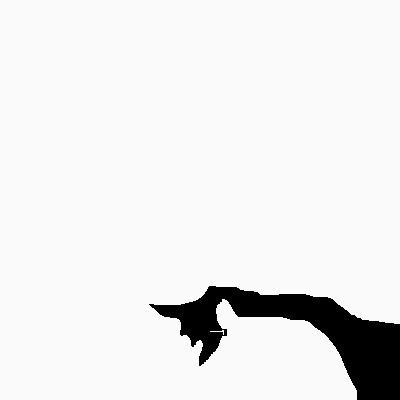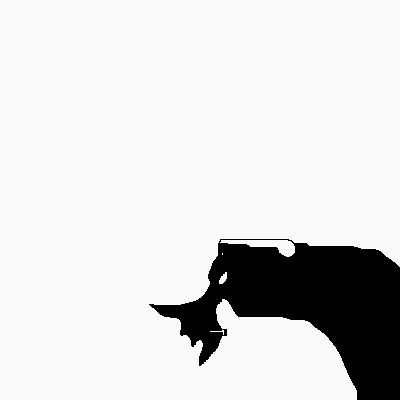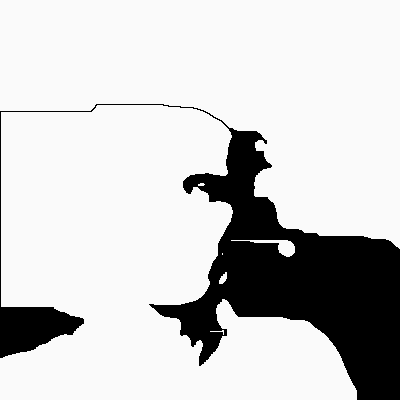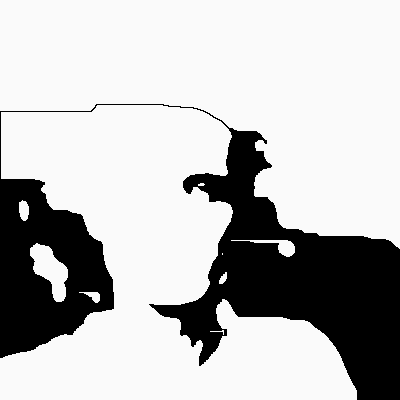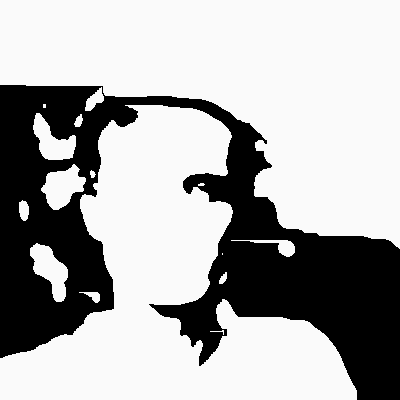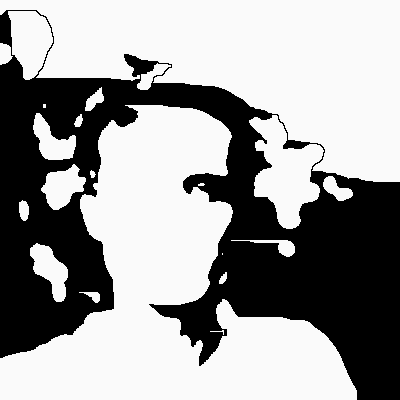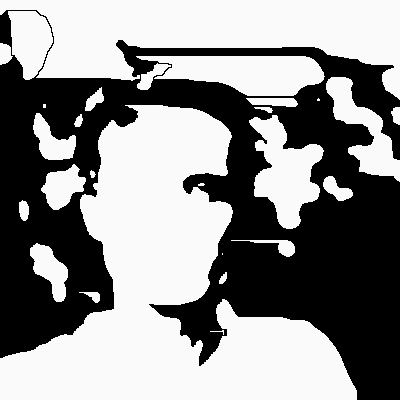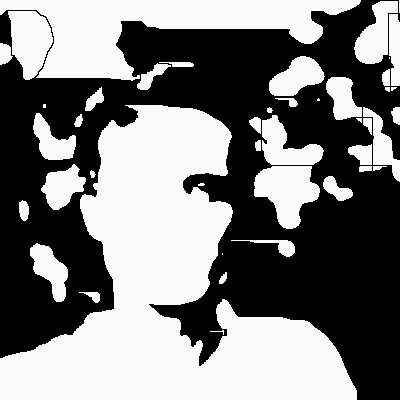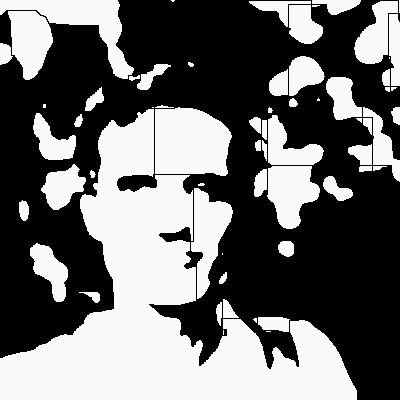## Validation

To check that the directions make sense, let's make the turtle run:

import matplotlib.pyplot as plt

xl=[]
yl=[]
x=0
y=0
di=0
with open("face.number") as fd:
while True:
if not char:
break
r=int(char)
if di==0:
x+=1
elif di==1:
y+=1
elif di==2:
x-=1
elif di==3:
y-=1
xl.append(x)
yl.append(y)
di = (di+r)%4
plt.plot(xl, yl)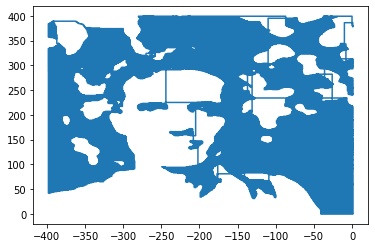This was good enough and I sent the number to Matt.

## Plotted

I was happy that Matt decided to feed the number to his pen plotter. The result on paper looks even better. Thank you Matt for creating these fascinating animations and explaining how you made them.